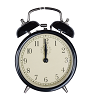You may also likeOn Time

On a clock the three hands - the second, minute and hour hands - are on the same axis. How often in a 24 hour day will the second hand be parallel to either of the two other hands?Estimating Angles

How good are you at estimating angles?LOGO Challenge 1 - Star Square

Can you use LOGO to create this star pattern made from squares. Only basic LOGO knowledge needed.

Two Exterior Triangles

Age 11 to 14 ShortChallenge Level

A square is labelled clockwise $ABCD$. $P$ and $Q$ are points outside the square such that triangles $ABP$ and $BCQ$ are both equilateral.

How big is angle $PQB$?

If you liked this problem, here is an NRICH task which challenges you to use similar mathematical ideas.

This problem is taken from the UKMT Mathematical Challenges.
You can find more short problems, arranged by curriculum topic, in our short problems collection.